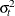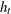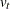# The AUTOREG Procedure

### OUTPUT Statement

• OUTPUT <OUT=SAS-data-set> <options> <keyword=name>;

The OUTPUT statement creates an output SAS data set as specified by the following options.

OUT=SAS-data-set

names the output SAS data set to contain the predicted and transformed values. If the OUT= option is not specified, the new data set is named according to the DATAn convention.

You can specify any of the following options.

ALPHACLI=number

sets the confidence limit size for the estimates of future values of the response time series. The ALPHACLI= value must be between 0 and 1. The resulting confidence interval has 1–number confidence. The default is ALPHACLI=0.05, which corresponds to a 95% confidence interval.

ALPHACLM=number

sets the confidence limit size for the estimates of the structural or regression part of the model. The ALPHACLM= value must be between 0 and 1. The resulting confidence interval has 1–number confidence. The default is ALPHACLM=0.05, which corresponds to a 95% confidence interval.

ALPHACSM=0.01 | 0.05 | 0.10

specifies the significance level for the upper and lower bounds of the CUSUM and CUSUMSQ statistics output by the CUSUMLB=, CUSUMUB=, CUSUMSQLB=, and CUSUMSQUB= options. The significance level specified by the ALPHACSM= option can be 0.01, 0.05, or 0.10. Other values are not supported.

You can specify the following values for keyword=name, where keyword specifies the statistic to include in the output data set and name gives the name of the variable in the OUT= data set to contain the statistic.

BLUS=variable

specifies the name of a variable to contain the values of the Theil’s BLUS residuals. Refer to Theil (1971) for more information on BLUS residuals.

CEV=variable
HT=variable

writes to the output data set the value of the error variancefrom the heteroscedasticity model specified by the HETERO statement or the value of the conditional error varianceby the GARCH= option in the MODEL statement.

CPEV=variable

writes the conditional prediction error variance to the output data set. The value of conditional prediction error variance is equal to that of the conditional error variance when there are no autoregressive parameters. See the section Predicted Values for details.

CONSTANT=variable

writes the transformed intercept to the output data set. The details of the transformation are described in Computational Methods.

CUSUM=variable

specifies the name of a variable to contain the CUSUM statistics.

CUSUMSQ=variable

specifies the name of a variable to contain the CUSUMSQ statistics.

CUSUMUB=variable

specifies the name of a variable to contain the upper confidence bound for the CUSUM statistic.

CUSUMLB=variable

specifies the name of a variable to contain the lower confidence bound for the CUSUM statistic.

CUSUMSQUB=variable

specifies the name of a variable to contain the upper confidence bound for the CUSUMSQ statistic.

CUSUMSQLB=variable

specifies the name of a variable to contain the lower confidence bound for the CUSUMSQ statistic.

LCL=name

writes the lower confidence limit for the predicted value (specified in the PREDICTED= option) to the output data set. The size of the confidence interval is set by the ALPHACLI= option. See the section Predicted Values for details.

LCLM=name

writes the lower confidence limit for the structural predicted value (specified in the PREDICTEDM= option) to the output data set under the name given. The size of the confidence interval is set by the ALPHACLM= option.

PREDICTED=name
P=name

writes the predicted values to the output data set. These values are formed from both the structural and autoregressive parts of the model. See the section Predicted Values for details.

PREDICTEDM=name
PM=name

writes the structural predicted values to the output data set. These values are formed from only the structural part of the model. See the section Predicted Values for details.

RECPEV=variable

specifies the name of a variable to contain the part of the predictive error variance () that is used to compute the recursive residuals.

RECRES=variable

specifies the name of a variable to contain recursive residuals. The recursive residuals are used to compute the CUSUM and CUSUMSQ statistics.

RESIDUAL=name
R=name

writes the residuals from the predicted values based on both the structural and time series parts of the model to the output data set.

RESIDUALM=name
RM=name

writes the residuals from the structural prediction to the output data set.

TRANSFORM=variables

transforms the specified variables from the input data set by the autoregressive model and writes the transformed variables to the output data set. The details of the transformation are described in the section Computational Methods. If you need to reproduce the data suitable for re-estimation, you must also transform an intercept variable. To do this, transform a variable that is all 1s or use the CONSTANT= option.

UCL=name

writes the upper confidence limit for the predicted value (specified in the PREDICTED= option) to the output data set. The size of the confidence interval is set by the ALPHACLI= option. For more information, see the section Predicted Values.

UCLM=name

writes the upper confidence limit for the structural predicted value (specified in the PREDICTEDM= option) to the output data set. The size of the confidence interval is set by the ALPHACLM= option.